### 《程序员》：增强学习在无人驾驶中的应用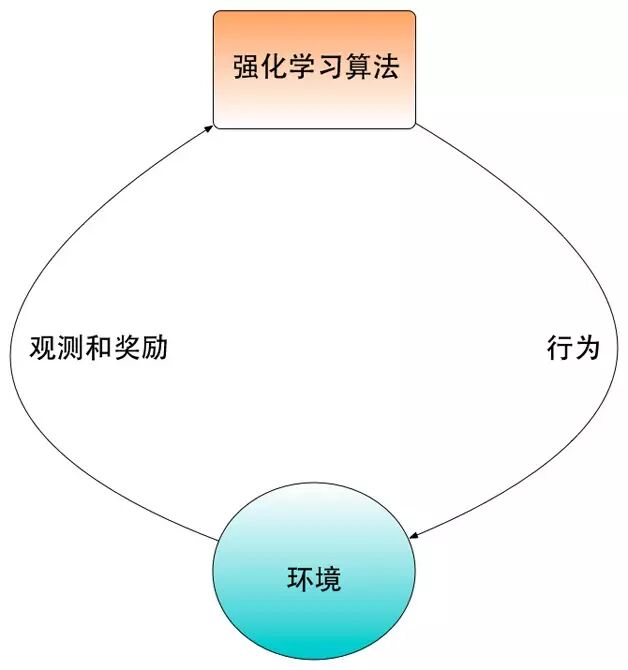ρ(π)=E{∑t=1∞γt−1rt|s0,π （1）

Qπ(s,a)=E{∑k=1∞γk−1rr+k|st=s,at=a,π}=Es′[r+γQpi(s′,a′)|s,a,π] （2）

➤REINFORCE

REINFORCE是最简单的reinforcement learning算法。其基本思想是通过在环境里面执行当前的策略直到一个回合结束（比如游戏结束），根据得到的奖励可以计算出当前策略的梯度。我们可以用这个梯度更新当前的策略得到新策略。在下面的回合，我们再用新的策略重复这个过程，一直到计算出的梯度足够小为止。最后得到的策略就是最优策略。

∇θρ(π)=∑t=1T▽θlogπ(at|st;θ)Rt （3）

REINFORCE的核心思想是通过从环境中获得的奖励判断执行行为的好坏。如果一个行为执行之后获得的奖励比较高，那么算出的梯度也会比较高，这样在更新后的策略中该行为被采样到的概率也会比较高。反之，对于执行之后获得奖励比较低的行为，因为计算出的梯度低，更新后的策略中该行为被采样到的概率也会比较低。通过在这个环境中反复执行各种行为，REIFORCE可以大致准确地估计出各个行为的正确梯度，从而对策略中各个行为的采样概率做出相应调整。

➤Deep Q-learning

Deep Q-learning是一种基于Q函数的增强学习算法。该算法对于复杂的每步行为之间存在较强的相关性环境有很好的效果。Deep Q-learning学习算法的基础是Bellman公式。我们在前面的章节已经介绍了Q函数的定义，如下所示。

Qpi(s,a)=E{∑k=1∞γk-1rr+k|st=s,at=a,π}=Es’[r+γQpi(s’,a’)|s,a,π] （4）

Q*(s,a)=Es’[r+γmaxa’Q*(s,a)|s,a] （5）

L(w)=E[(r+γmaxa’Q*(s’,a’;w)-Q(s,a;w))2]（6）

∂L(w)∂w=E[(r+γmaxa’Q*(s’,a’;w)-Q(s,a;w))∂Q(s,a;w∂w) （7）

r+γmaxa’Q*(s’,a’;w-) （8）

Q-learning算法的框图如图2所示。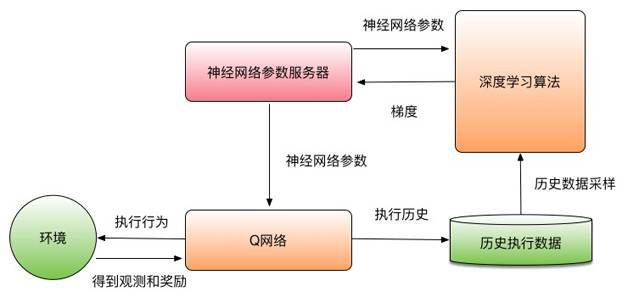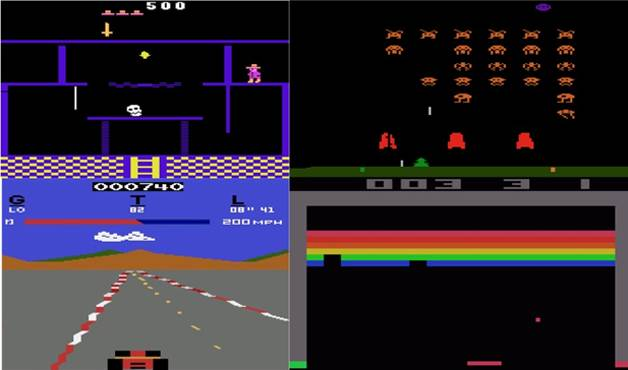Deep Q-learning算法在没有任何额外知识的情况下，完全以图像和获得的奖励进行输入。在大部分Atari游戏中都大大超过了人类性能。这是深度学习或者增强学习出现前完全不可能完成的任务。Atari游戏是第一个Deep Q-learning解决了用其他算法都无法解决的问题，充分显示了将深度学习和增强学习结合的优越性和前景。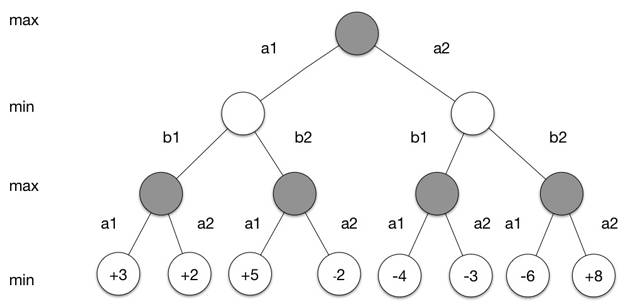vπ(s)=Eπ[Gt|St=s] （9）

v*(s)=maxπ1minπ2vπ(s) （10）

▽w=α(Gt-v(st;w))▽wv(st;w) （11）

▽w=α(v(st+1;w)-v(st;w))▽wv(st;w)（12）

➤自动驾驶模拟器

➤增强学习在自动驾驶中的应用和展望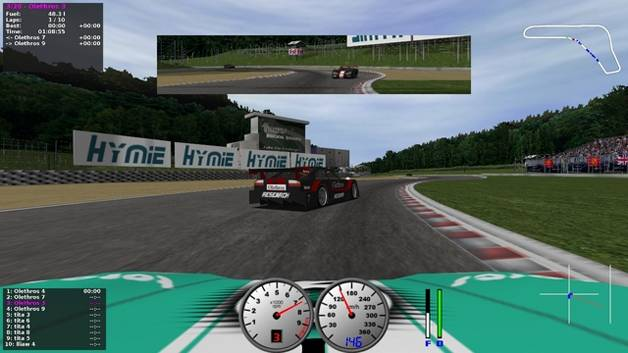﻿